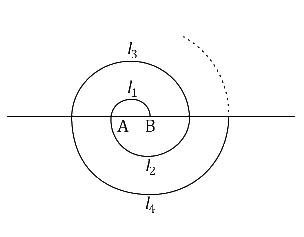# Ex.5.3 Q18 Arithmetic Progressions Solution - NCERT Maths Class 10

## Question

A spiral is made up of successive semicircles, with centres alternately at $$A$$ and $$B,$$ starting with centre at $$A$$ of radii $$0.5,$$ $$1.0 \,\rm{cm}, 1.5 \,\rm{cm}, 2.0 \,\rm{cm}, .........$$as shown in figure. What is the total length of such a spiral made up of thirteen consecutive semicircles?

\begin{align}\left[ {{\rm{Take}}\,\,\pi = \frac{{22}}{7}} \right] \end{align}Video Solution
Arithmetic Progressions
Ex 5.3 | Question 18

## Text Solution

What is Known?

Radii of the $$13$$ semicircles.

What is Unknown?

Length of the spiral made.

Reasoning:

Sum of the first $$n$$terms of an AP is given by $${S_n} = \frac{n}{2}\left[ {2a + \left( {n - 1} \right)d} \right]$$

Where $$a$$ is the first term, $$d$$is the common difference and $$n$$ is the number of terms.

Steps:

Semi-perimeter of circle, $$l = \pi r$$

\begin{align}{{l}_{1}}& =\pi \times \left( 0.5\,\,\text{cm} \right)=0.5\pi \,\,\text{cm } \\ {{l}_{2}}&=\pi \times \left( 1\,\,\text{cm} \right)=\pi \,\,\text{cm} \\ {{l}_{3}} &=\pi \times \left( 1.5\,\,\text{cm} \right)=1.5\pi \,\text{cm} \\\end{align}

Therefore, $${l_1},{\rm{ }}{l_2},{\rm{ }}{l_3},$$ i.e. the lengths of the semi-circles are in an A.P.,

\begin{align}&0.5\pi ,\pi ,1.5\pi ,2\pi\dots\dots\dots\\&a = 0.5\pi \\&d = \pi - 0.5\pi = 0.5\pi\end{align}

We know that the sum of $$n$$ terms of an A.P. is given by

\begin{align}{S_n} &= \frac{n}{2}\left[ {2a + \left( {n - 1} \right)d} \right]\\{S_{13}} &\!=\!\frac{{13}}{2}\left[ {2 \!\times\! \left( {0.5\pi } \right)\!+\!\left( {13\!-\!1} \right)\left( {0.5\pi } \right)}\!\right]\\ &= \frac{{13}}{2}\left[ {\pi + 6\pi } \right]\\ &= \frac{{13}}{2} \times 7\pi \\ &= \frac{{13}}{2} \times 7 \times \frac{{22}}{7}\\ &= 143\end{align}

Therefore, the length of such spiral of thirteen consecutive semi-circles will be $$143\, \rm{cm.}$$

Learn from the best math teachers and top your exams

• Live one on one classroom and doubt clearing
• Practice worksheets in and after class for conceptual clarity
• Personalized curriculum to keep up with school